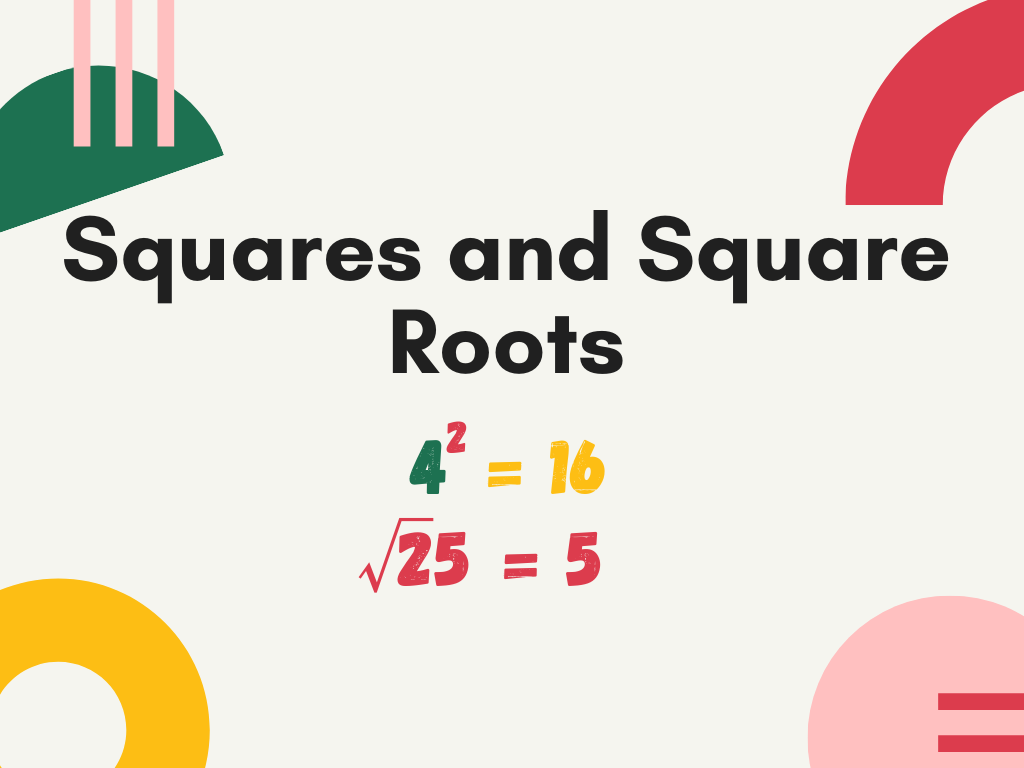## Squares and Square Roots Class 8th

Introduction In geometry, we know that a square is a figure enclosed by four equal line segments and the area of the square is given by side×side (where ‘side’ indicates the length of each side of the square). Similarly, in the number system, we find the square of the numbers with the help of this …